# Cylinder - math word problems

1. ContainerThe container has a cylindrical shape the base diameter 0.8 m and the area of the base is equal to the area of the wall. How many liters of water can we pour into the container?
2. Water flowHow much water flow in pipe with a diameter of 16 cm in 1 hour if the water velocity is 2.5 m/s?
3. DiameterWhat is the inside diameter of the cylinder container and if half a liter of water reaches a height 15 cm?
4. Megapascals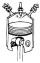What is the area of crosssection of the piston, if the force of 300 kN produces a pressure of 5 MPa?
5. Rope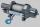How many meters of rope 10 mm thick will fit on the bobbin diameter of 200 mm and length 350 mm (central mandrel have a diameter 50 mm)?
6. Fuel economyHow many kilometers is sufficient petrol in the cylinder fuel tank with a diameter 40 cm and the base of tank length 1 m, when it is filled to 60% and if the car consume 15 liters per 100 km?
7. Hexagon rotationA regular hexagon of side 6 cm is rotated through 60° along a line passing through its longest diagonal. What is the volume of the figure thus generated?
8. Surface area of cylinder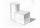Determine the lateral surface of the rotary cylinder which is circumscribed cube with edge length 5 cm.
9. VintnerHow high can vintner fill keg with crushed red grapes if these grapes occupy a volume of 20 percent? Keg is cylindrical with a diameter of the base 1 m and a volume 9.42 hl. Start from the premise that says that fermentation will fill the keg (the number
10. Horizontal Cylindrical SegmentHow much fuel is in the tank of horizontal cylindrical segment with a length 10m, width of level 1 meter and level is 0.2 meters below the upper side of the tank?
11. PoolThe pool has the shape of a cylinder with a diameter 4.6m, the presumed height is 130cm. The bucket fits 12l of water. How many buckets do you have to bring to fill this pool?
12. Copper wire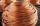What is the weight of 1000 m copper wire with a diameter of 5 mm when metric density p = 8.8 g/cm3?
13. Rotary bodiesThe rotating cone and the rotary cylinder have the same volume 180 cm3 and the same height v = 15 cm. Which of these two bodies has a larger surface area?
14. Cylindrical tankCylindrical tank holds 600hl water and is deep 2.5 m. Calculate the diameter of the cylinder.
15. Water reservoir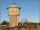The reservoir shape of a cylinder having a diameter 8 m and a height 2.8 m. The device indicates that the reservoir contains 800 hectoliters of water. Calculate how much % of volume is used.
16. Water tankThe water tank has a cylindrical shape with a base diameter of 4.2 m and is 80 cm deep. How many minutes will take fill it 10 cm below the edge of the tank if water flowing 2 liters per second?
17. Gasoline tank cylindrical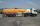What is the inner diameter of the tank, which is 8 m long and contains 40 cubic cubic meters of gasoline?
18. The cylinder baseThe cylinder with a base of 8 dm2 has a volume of 120 liters. From a cylinder fully filled with water, 40 liters of water was removed. At what height from the bottom /with precision to dm/ is the water level?
19. Cylinder twiceIf the radius of the cylinder increases twice, and the height is reduced twice, then the volume of the cylinder increases (how many times?):
20. CylinderIn a 1-meter diameter cylinder is 1413 liters of water, which is 60% of the cylinder. Calculate the cylinder height in meters, do not write the units. The resulting value round and write as an integer.

Do you have an interesting mathematical word problem that you can't solve it? Submit math problem, and we can try to solve it.

We will send a solution to your e-mail address. Solved examples are also published here. Please enter the e-mail correctly and check whether you don't have a full mailbox.

Please do not submit problems from current active competitions such as Mathematical Olympiad, correspondence seminars etc...